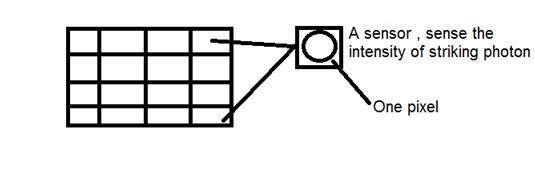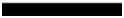# Concept of Pixel

## Pixel

Pixel is the smallest element of an image. Each pixel correspond to any one value. In an 8-bit gray scale image, the value of the pixel between 0 and 255. The value of a pixel at any point correspond to the intensity of the light photons striking at that point. Each pixel store a value proportional to the light intensity at that particular location.

### PEL

A pixel is also known as PEL. You can have more understanding of the pixel from the pictures given below.

In the above picture, there may be thousands of pixels, that together make up this image. We will zoom that image to the extent that we are able to see some pixels division. It is shown in the image below.In the above picture, there may be thousands of pixels, that together make up this image. We will zoom that image to the extent that we are able to see some pixels division. It is shown in the image below.## Relation ship with CCD array

We have seen that how an image is formed in the CCD array. So a pixel can also be defined as

The smallest division the CCD array is also known as pixel.

Each division of CCD array contains the value against the intensity of the photon striking to it. This value can also be called as a pixel### Calculation of total number of pixels

We have define an image as a two dimensional signal or matrix. Then in that case the number of PEL would be equal to the number of rows multiply with number of columns.

This can be mathematically represented as below:

Total number of pixels = number of rows ( X ) number of columns

Or we can say that the number of (x,y) coordinate pairs make up the total number of pixels.

We will look in more detail in the tutorial of image types , that how do we calculate the pixels in a color image.

## Gray level

The value of the pixel at any point denotes the intensity of image at that location , and that is also known as gray level.

We will see in more detail about the value of the pixels in the image storage and bits per pixel tutorial, but for now we will just look at the concept of only one pixel value.

### Pixel value.(0)

As it has already been define in the beginning of this tutorial , that each pixel can have only one value and each value denotes the intensity of light at that point of the image.

We will now look at a very unique value 0. The value 0 means absence of light. It means that 0 denotes dark, and it further means that when ever a pixel has a value of 0, it means at that point , black color would be formed.

Have a look at this image matrix

 0 0 0 0 0 0 0 0 0

Now this image matrix has all filled up with 0. All the pixels have a value of 0. If we were to calculate the total number of pixels form this matrix , this is how we are going to do it.

Total no of pixels = total no. of rows X total no. of columns

= 3 X 3

= 9.

It means that an image would be formed with 9 pixels, and that image would have a dimension of 3 rows and 3 column and most importantly that image would be black.

The resulting image that would be made would be something like thisNow why is this image all black. Because all the pixels in the image had a value of 0.